# Estimating the extrema of noisy curves and optimization using spline surface approximation

Estimating maxima and minima of a noisy curve turns out to be very hard and to a large part is still an open question. In this blog post we will discover some strategies to encounter the problem. Another interpretation is given in the context of optimization. Consider an optimization problem where the (smooth) objective function is only evaluated at some, maybe random and noisy, points or due to computational issues can only be sparsely evaluated. Then this algorithm will give a fast estimate of the optimal values.

## 1. Estimation based on Splines

We assume that a smooth at least two times differentiable curve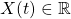is observed at independent randomly-distributed pointscontaminated with additional noise. Our model is then given by

(1)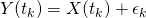where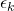are i.i.d. random variables with,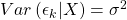andis independent of. Our goal is to determine allsuch that the derivative

(2)### 1.1 Natural cubic splines

Consider the following minimization problem, among all functions with two continuous derivatives find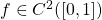such that

(3)is minimized. whereis a fixed smoothing parameter and. It can be shown , that (3) has an unique minimizer which is a particular natural cubic spline. More generally a natural cubic spline with knotsis a piecewise polynomial of order 4, with additional constrains such that the the function is linear beyond the boundary knots, represented withBasis functions. When the knots are given bythe natural cubic spines solves (3) in that case an optimal solution for 3 is given by.

Letthe solution to the minimization problem usingis thus given by

(4)A popular basis choice is derived from the truncated power series and given by

(5)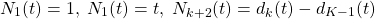where

(6)We will use this basis as illustration for our method from here on. An important inside is that for a fixed interval limited by the nodes,can be represented using a cubic function (see ). In particular

(7)The figure shows an example with the grey dots show the observed Y while the black dots indicate the estimated extrema by the algorithm. The spline (brown curve) is estimated using K=5, the rainbow colored curves are the representation of the spline using a polynomial of order 3 between a sector delimited by two neighbor knots.

Accordingly,

(8)For, the real possible extremaof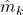are thus given by

(9)this will then be an extrema ofifand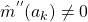.

### 1.2 Estimation and Asymptotic

To estimate the extrema we replace the spline coefficientsby, their estimated counterpart, as specified in (4) to deriveandaccording to (8) and (9). First verify that for fixed,(10)and

(11)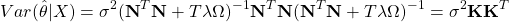therefore

(12)(13)(14)where,,.

After using a Taylor (see here for example) expansion and some calculus we can derive

(15)The first order Taylor expansion of the variance is given by

(16)where

(17)(18)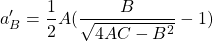(19)(20)## 2. White noise representation

The white noise version, as descibed in , of (1) is

(21)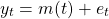where the noise term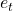can be interpreted asandis the error variance when the design point equals, andcan be figuratively represented as a ‘derivative’ of standard Brownian motion, in the sense
that.

In the white noise case, the corresponding equivalent basis to is given bywith the knot densitythen

(22)Then the continuous analogue to (8) is given by

(23)(24)(25)Accordingly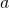is an extrema ofif and only if

(26)therefore an extrema is observed ifis a fix point.P. Craven and G. Wahba, “Smoothing noisy data with spline functions,” Numer. math., vol. 31, iss. 4, p. 377–403, 1978.
[Bibtex]
@article{Wahba1979,
author = {Craven, Peter and Wahba, Grace},
title = {Smoothing Noisy Data with Spline Functions},
year = {1978},
issue_date = {December 1978},
publisher = {Springer-Verlag},
volume = {31},
number = {4},
issn = {0029-599X},
url = {https://doi.org/10.1007/BF01404567},
doi = {10.1007/BF01404567},
abstract = {Smoothing splines are well known to provide nice curves which smooth discrete, noisy data. We obtain a practical, effective method for estimating the optimum amount of smoothing from the data. Derivatives can be estimated from the data by differentiating the resulting (nearly) optimally smoothed spline.We consider the model y i ( t i )+ i , i =1, 2, ..., n , t i [0, 1], where g W 2 ( m ) ={ f : f , f , ..., f ( m 1) abs. cont., f ( m ) 2[0,1]}, and the { i } are random errors with E i =0, E i j = 2 ij . The error variance 2 may be unknown. As an estimate of g we take the solution g n, to the problem: Find f W 2 (m) to minimize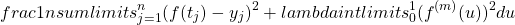. The function g n, is a smoothing polynomial spline of degree 2 m 1. The parameter controls the tradeoff between the "roughness" of the solution, as measured by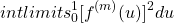, and the infidelity to the data as measured by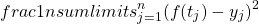, and so governs the average square error R( ; g)=R( ) defined by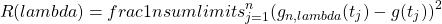. We provide an estimate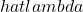, called the generalized cross-validation estimate, for the minimizer of R( ) . The estimateis the minimizer of V ( ) defined by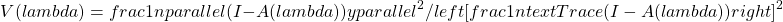, where y=(y 1, ..., y n)t and A ( ) is the n n matrix satisfying (g n, ( t 1), ..., g n, ( t n))t= A ( ) y . We prove that there exist a sequence of minimizers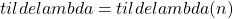of EV( ) , such that as the (regular) mesh {t i} i=1 n becomes finer,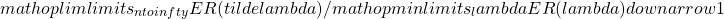. A Monte Carlo experiment with several smooth g 's was tried with m =2, n =50 and several values of 2, and typical values of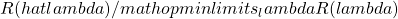were found to be in the range 1.01---1.4. The derivative g of g can be estimated by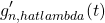. In the Monte Carlo examples tried, the minimizer of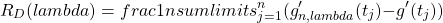tended to be close to the minimizer of R(¿) , so thatwas also a good value of the smoothing parameter for estimating the derivative.},
journal = {Numer. Math.},
month = {dec},
pages = {377–403},
numpages = {27},
keywords = {MOS:65D10, MOS:65D25, CR:5.17}
}
 C. REINSCH, “Smoothing by spline functions.,” Numerische mathematik, vol. 10, pp. 177-183, 1967.
[Bibtex]
@article{Reinsch1967,
author = {REINSCH, CH.H.},
journal = {Numerische Mathematik},
keywords = {numerical analysis},
pages = {177-183},
title = {Smoothing by Spline Functions.},
url = {http://eudml.org/doc/131782},
volume = {10},
year = {1967},
}
 P. Hall and J. D. Opsomer, “Theory for penalised spline regression,” Biometrika, vol. 92, iss. 1, p. 105–118, 2005.
[Bibtex]
@article{Hall92,
ISSN = {00063444},
URL = {http://www.jstor.org/stable/20441169},
abstract = {Penalised spline regression is a popular new approach to smoothing, but its theoretical properties are not yet well understood. In this paper, mean squared error expressions and consistency results are derived by using a white-noise model representation for the estimator. The effect of the penalty on the bias and variance of the estimator is discussed, both for general splines and for the case of polynomial splines. The penalised spline regression estimator is shown to achieve the optimal nonparametric convergence rate established by Stone (1982).},
author = {Peter Hall and J. D. Opsomer},
journal = {Biometrika},
number = {1},
pages = {105--118},
publisher = {[Oxford University Press, Biometrika Trust]},
title = {Theory for Penalised Spline Regression},
urldate = {2022-04-16},
volume = {92},
year = {2005}
}

This site uses Akismet to reduce spam. Learn how your comment data is processed.